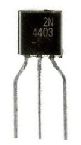﻿ How to Connect a Transistor in a Circuit for Current Amplification# How to Connect a Transistor in a Circuit for Current AmplificationIn this circuit, we will show how to connect a transistor in a circuit for current amplification.

Transistors are devices that can amplify a signal in a circuit. If a signal in a circuit is too weak, it may not be enough to provide power to turn or or drive the load for a circuit. A transistor can provide amplfication from anywhere to up to 10,000% gain so that it can boost a signal in a circuit up to a desired level.

Say, we have a circuit in which we want to light an LED, but the current in the circuit is 40µA, too small to light an LED. If this is the current that we have flowing through the circuit, it's too small to do the desired task, which is to light the LED. However, if we place a transistor in the circuit, which has a gain of 100, the signal will be boosted from 40µA to 40x100=4mA. This current will now be sufficient to light our LED in the circuit. So transistor amplification has tremendous application when we need signal gain.

Below is the schematic for the transistor. The transistor used is a bipolar NPN transistor.This is can be seen as the more real life like model of the above schematic.In this circuit, a small current travels from the voltage source of the circuit. This current is not large enough to light the LED. If this circuit didn't have a transistor to amplify the current, the LED would not light. However, with the transistor and the amplification it provides, the current is amplified large enough to light the LED.

This is how it works:
-The small current travels from the voltage source into the base of the transistor. A small current at the base turns on the transistor.
-The current is then amplified and travels from the emitter of the transistor to the collector. The amplified current is large enough to turn on and light the LED.
-A separate power source must be connected to the collector of the transistor because a transistor is an active device, which means it needs power in order to operate. Check the power requirements of the transistor in use.

So now you know how to connect a bipolar NPN transistor for amplification.

Related Resources